# Fission and FusionPage 3

#### WATCH ALL SLIDES

Slide 25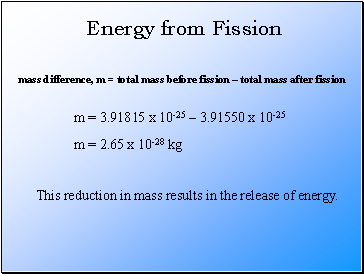Energy from Fission

mass difference, m = total mass before fission – total mass after fission

m = 3.91815 x 10-25 – 3.91550 x 10-25

m = 2.65 x 10-28 kg

This reduction in mass results in the release of energy.

Slide 26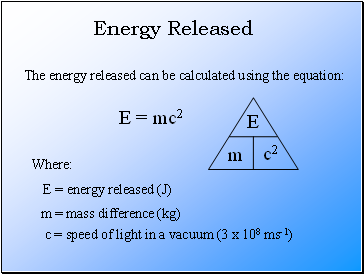## Energy Released

The energy released can be calculated using the equation:

E = mc2

Where:

E = energy released (J)

m = mass difference (kg)

c = speed of light in a vacuum (3 x 108 ms-1)

Slide 27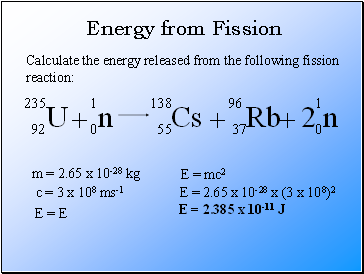Energy from Fission

E = mc2

Calculate the energy released from the following fission reaction:

m = 2.65 x 10-28 kg

c = 3 x 108 ms-1

E = E

E = 2.65 x 10-28 x (3 x 108)2

E = 2.385 x 10-11 J

Slide 28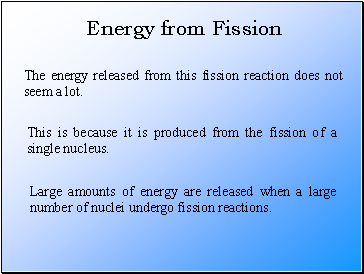Energy from Fission

The energy released from this fission reaction does not seem a lot.

This is because it is produced from the fission of a single nucleus.

Large amounts of energy are released when a large number of nuclei undergo fission reactions.

Slide 29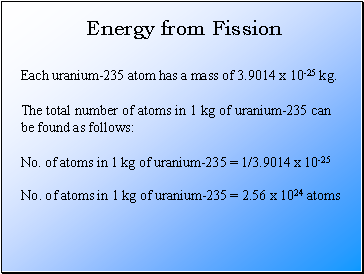Energy from Fission

Each uranium-235 atom has a mass of 3.9014 x 10-25 kg.

The total number of atoms in 1 kg of uranium-235 can be found as follows:

No. of atoms in 1 kg of uranium-235 = 1/3.9014 x 10-25

No. of atoms in 1 kg of uranium-235 = 2.56 x 1024 atoms

Slide 30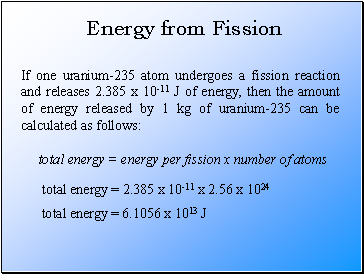Energy from Fission

If one uranium-235 atom undergoes a fission reaction and releases 2.385 x 10-11 J of energy, then the amount of energy released by 1 kg of uranium-235 can be calculated as follows:

total energy = energy per fission x number of atoms

total energy = 2.385 x 10-11 x 2.56 x 1024

total energy = 6.1056 x 1013 J

Slide 31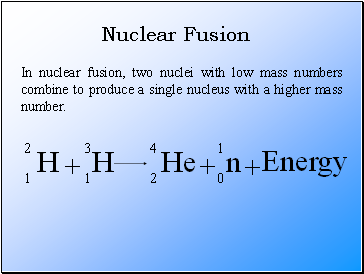## Nuclear Fusion

In nuclear fusion, two nuclei with low mass numbers combine to produce a single nucleus with a higher mass number.

Slide 32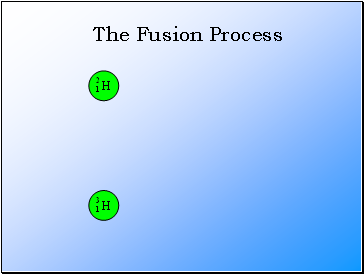The Fusion Process

Slide 33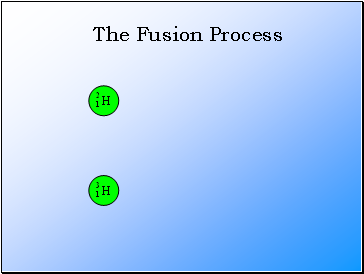The Fusion Process

Slide 34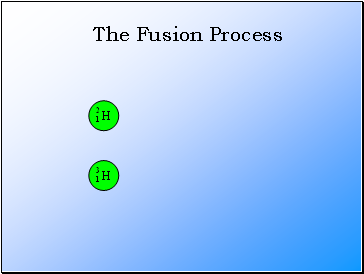The Fusion Process

Slide 35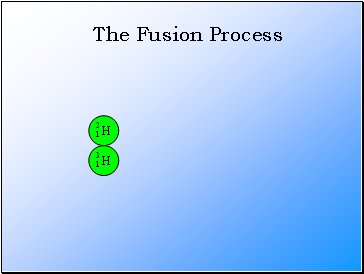The Fusion Process

Slide 36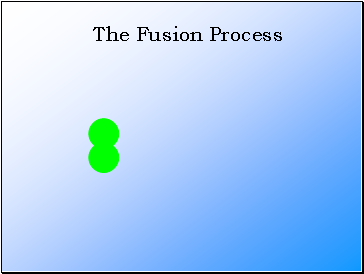Go to page:
1  2  3  4